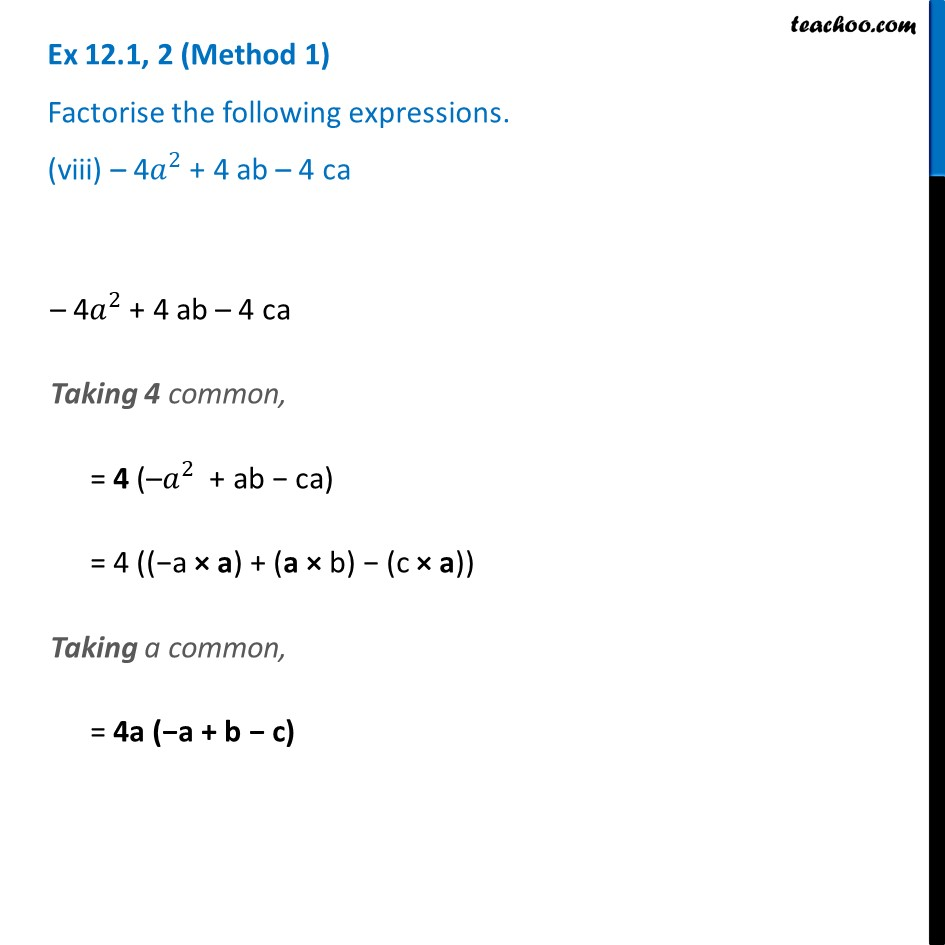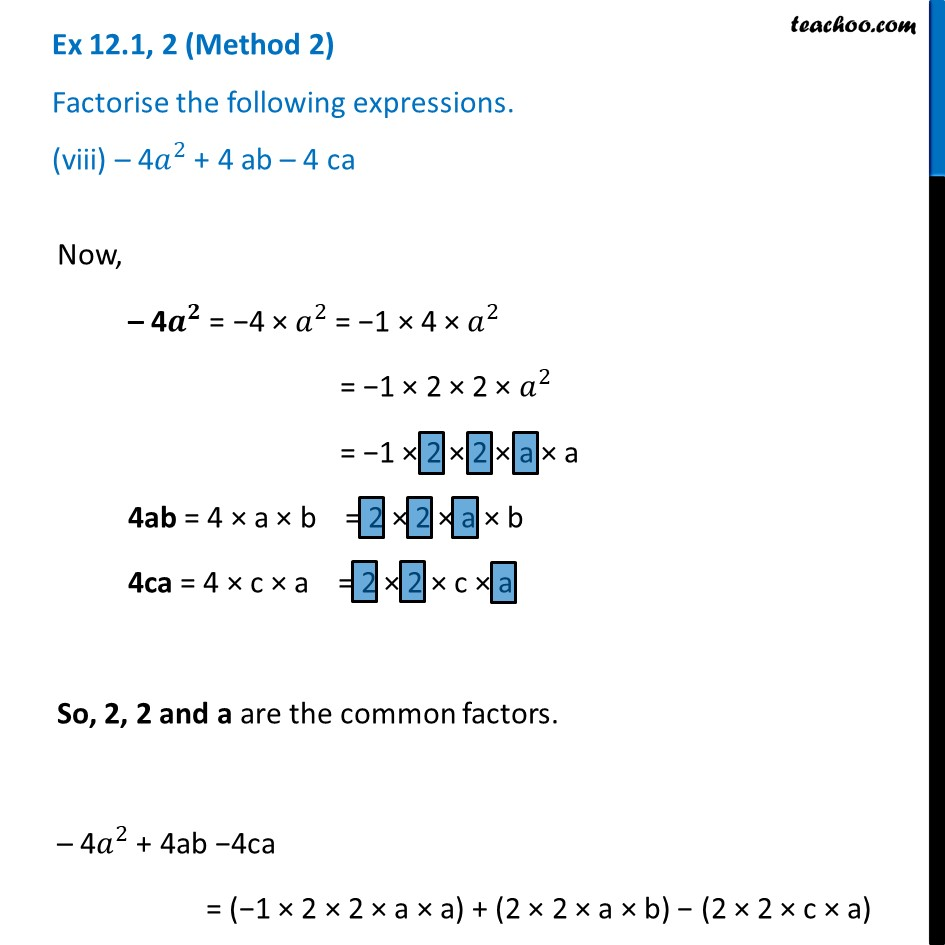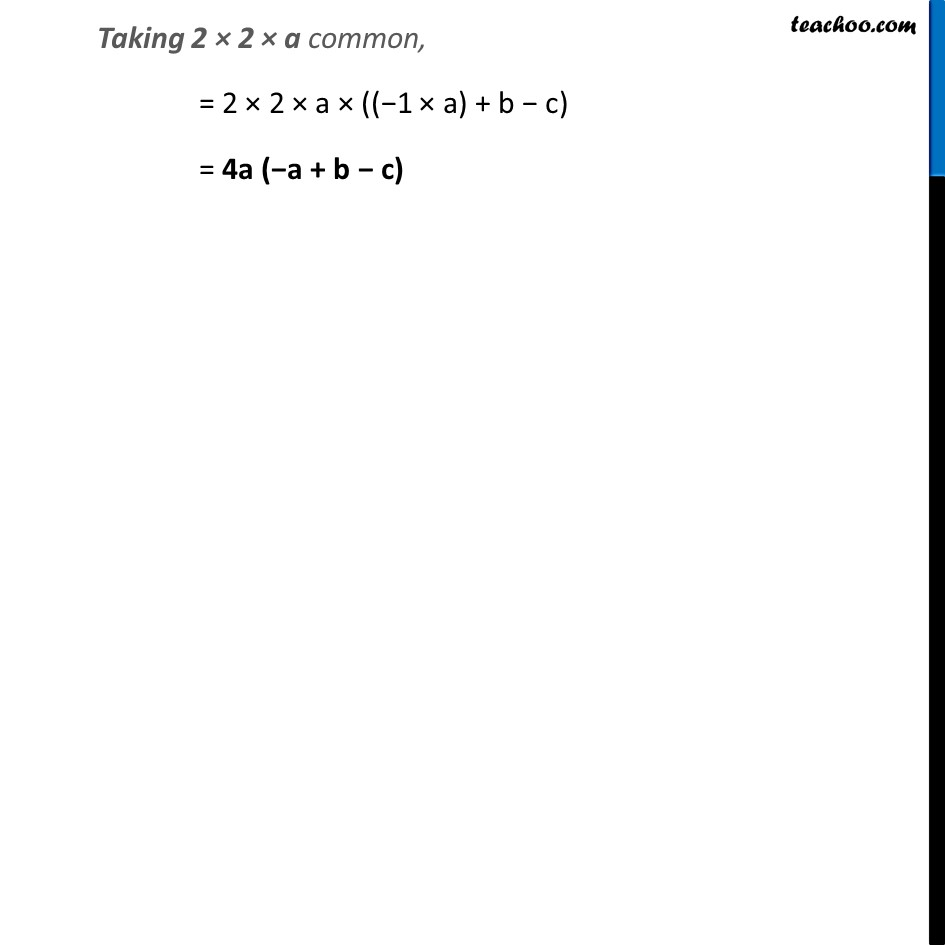Ex 12.1

Chapter 12 Class 8 Factorisation
Serial order wiseLearn in your speed, with individual attention - Teachoo Maths 1-on-1 Class

### Transcript

Ex 12.1, 2 (Method 1) Factorise the following expressions. (viii) – 4𝑎^2 + 4 ab – 4 ca– 4𝑎^2 + 4 ab – 4 ca Taking 4 common, = 4 (–𝑎^2 + ab − ca) = 4 ((−a × a) + (a × b) − (c × a)) Taking a common, = 4a (−a + b − c) Ex 12.1, 2 (Method 2) Factorise the following expressions. (viii) – 4𝑎^2 + 4 ab – 4 caNow, – 4𝒂^𝟐 = −4 × 𝑎^2 = −1 × 4 × 𝑎^2 = −1 × 2 × 2 × 𝑎^2 = −1 × 2 × 2 × a × a 4ab = 4 × a × b = 2 × 2 × a × b 4ca = 4 × c × a = 2 × 2 × c × a So, 2, 2 and a are the common factors. – 4𝑎^2 + 4ab −4ca = (−1 × 2 × 2 × a × a) + (2 × 2 × a × b) − (2 × 2 × c × a) Taking 2 × 2 × a common, = 2 × 2 × a × ((−1 × a) + b − c) = 4a (−a + b − c)